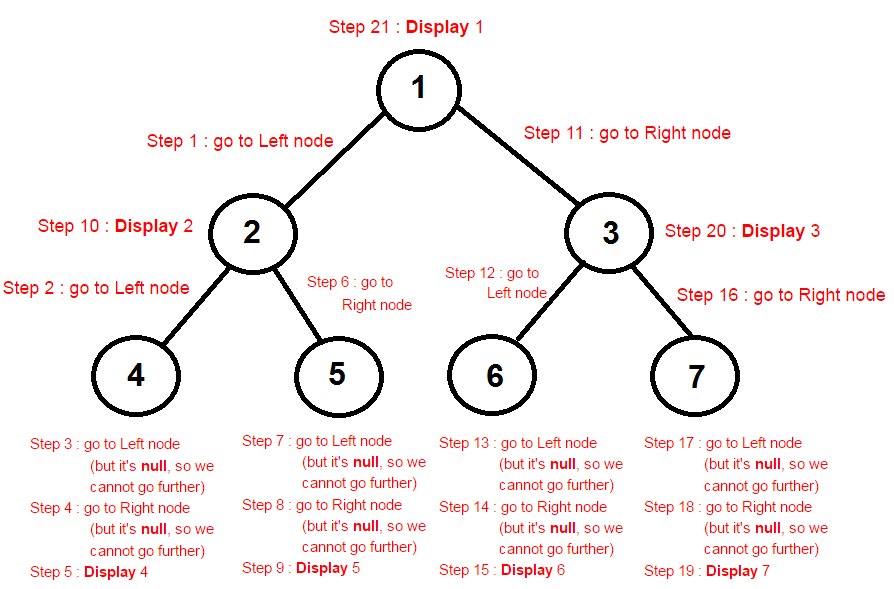### PostOrder traversal of Binary Tree in java

In this data structure tutorial we will learn PostOrder traversal of Binary Tree in java with program and examples.
Contents of page >
1. What happens in PostOrder traversal of Binary Tree in java >
2. What are Steps in PostOrder Traversal of Binary Tree in java >
3. Diagram of PostOrder traversal of Binary Tree in java >
Result of PostOrder traversal of Binary Tree in java is >
1. Logic for PostOrder traversal of Binary Tree in java >
2. Full program/ example to show PostOrder traversal of Binary Tree in java >

1. What happens in PostOrder traversal of Binary Tree in java >
In PostOrder traversal of Binary Tree in java each node is processed after traversing its subtree.

2. What are Steps in PostOrder Traversal of Binary Tree in java >
Steps in PostOrder Traversal of Binary Tree in java -
1. traverse left subtree
2. traverse the right subtree.
3. Visit the node.

Diagram of PostOrder traversal of Binary Tree in java >Result of PostOrder traversal of Binary Tree in java is >
4 5 2 6 7 3 1

4. Logic for PostOrder traversal of Binary Tree in java >
 /**    * This method will do Post-order Traversal of binary tree in java.    * Method will pass leftNode of binary tree recursively    * Once leftNode child is done, pass rightNode of binary tree recursively    */    public void postorderTraversalMethod(BinaryTreeNode binaryTreeNode) {           // Continue if binaryTreeNode is not null           if (binaryTreeNode != null) {                  // pass leftNode of binary tree recursively                  postorderTraversalMethod(binaryTreeNode.leftNode);                  // pass rightNode of binary tree recursively                  postorderTraversalMethod(binaryTreeNode.rightNode);                                   // Display data of current binaryTreeNode.                  System.out.print(binaryTreeNode.data + " ");           }    }

5. Full program/ example to show PostOrder traversal of Binary Tree in java >
 /** * www.JavaMadeSoEasy.com * Write a program to perform PostOrder Traversal of Binary Tree in java * * Class to perform PostOrder Traversal of Binary Tree in java */ public class PostOrderTraversalOfBinaryTree {       /**    * Create Node of Binary Tree    */    public static class BinaryTreeNode {           // Left and right node of Binary tree           BinaryTreeNode leftNode;           BinaryTreeNode rightNode;           // Data of BinaryTree           int data;           BinaryTreeNode(int data) {                  this.data = data;           }    }    /**    * This method will do Post-order Traversal of binary tree in java.    * Method will pass leftNode of binary tree recursively    * Once leftNode child is done, pass rightNode of binary tree recursively    */    public void postorderTraversalMethod(BinaryTreeNode binaryTreeNode) {           // Continue if binaryTreeNode is not null           if (binaryTreeNode != null) { // Visit the node-Printing the node data                  // pass leftNode of binary tree recursively                  postorderTraversalMethod(binaryTreeNode.leftNode);                  // pass rightNode of binary tree recursively                  postorderTraversalMethod(binaryTreeNode.rightNode);                                   // Display data of current binaryTreeNode.                  System.out.print(binaryTreeNode.data + " ");           }    }    /**    * Main method to perform PostOrder Traversal of Binary Tree in java    */    public static void main(String[] args) {           PostOrderTraversalOfBinaryTree postOrderTraversalOfBinaryTree =                                                new PostOrderTraversalOfBinaryTree();           // Now, let's start creating nodes of Binary tree           // create node1 (which is ROOT node)           BinaryTreeNode binaryTreeNode1 = new BinaryTreeNode(1);           // Left node of node1           BinaryTreeNode node2 = new BinaryTreeNode(2);           binaryTreeNode1.leftNode = node2;           // Right node of node1           BinaryTreeNode node3 = new BinaryTreeNode(3);           binaryTreeNode1.rightNode = node3;           // Left node of node2           BinaryTreeNode node4 = new BinaryTreeNode(4);           node2.leftNode = node4;           // Right node of node2           BinaryTreeNode node5 = new BinaryTreeNode(5);           node2.rightNode = node5;           // Left node of node3           BinaryTreeNode node6 = new BinaryTreeNode(6);           node3.leftNode = node6;           // Right node of node3           BinaryTreeNode node7 = new BinaryTreeNode(7);           node3.rightNode = node7;           System.out.println("Post order Traversal of Binary Tree in java : ");           // Pass root node to Post order traversal method           postOrderTraversalOfBinaryTree                        .postorderTraversalMethod(binaryTreeNode1);    } } /* Output Post order Traversal of Binary Tree in java : 4 5 2 6 7 3 1 */

Summary -
So in this data structure tutorial we learned In PostOrder traversal of Binary Tree in java each node is processed after traversing its subtree. Steps in PostOrder Traversal of Binary Tree in java. Diagram of PostOrder traversal of Binary Tree in java.Logic for PostOrder traversal of Binary Tree in java. Full program/ example to show PostOrder traversal of Binary Tree in java.

Having any doubt? or you liked the tutorial! Please comment in below section.